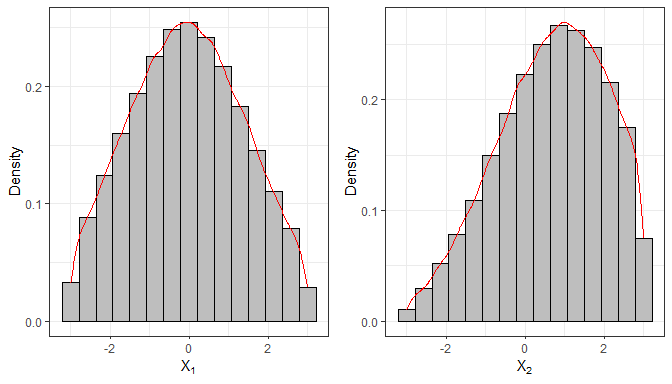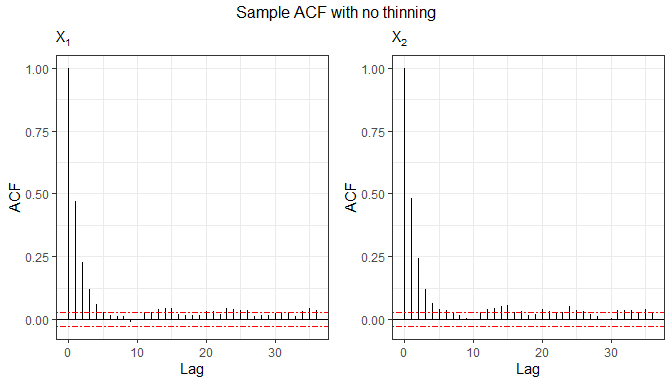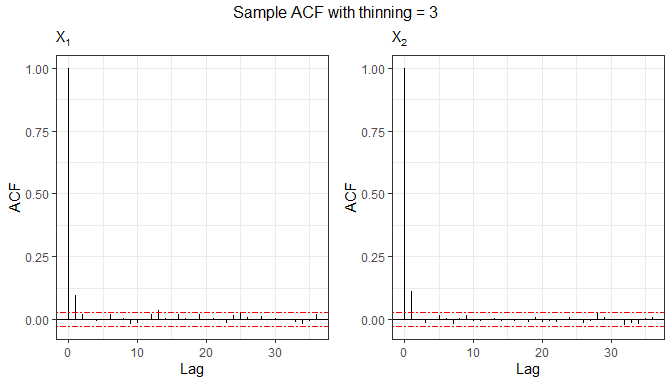## The `relliptical R` package

The `relliptical R` package offers random numbers generation from members of the truncated multivariate elliptical family of distribution such as the truncated versions of the Normal, Student-t, Pearson VII, Slash, Logistic, Kotz-type, among others. Particular distributions can be provided by specifying the density generating function. It also computes the first two moments (covariance matrix as well) for some particular distributions. For more details see (Valeriano, Galarza, and Matos 2021).

Next, we will show the functions available in the package.

## Sampling random numbers

The function `rtelliptical` generates observations from a truncated multivariate elliptical distribution with location parameter `mu`, scale matrix `Sigma`, lower and upper truncation points `lower` and `upper` via Slice Sampling algorithm (Neal 2003) with Gibbs sampler (Robert and Casella 2010) steps. The `dist` argument represents the truncated distribution to be used. The values are `Normal`, `t`, `PE`, `PVII`, `Slash`, and `CN`, for the truncated Normal, Student-t, Power Exponential, Pearson VII, Slash, and Contaminated Normal distributions, respectively.

In the following example, we generate n = 105 samples from the truncated bivariate Normal distribution.

``````library(relliptical)

# Sampling from the Truncated Normal distribution
set.seed(1234)
mu  = c(0, 1)
Sigma = matrix(c(3,0.6,0.6,3), 2, 2)
lower = c(-3, -3)
upper = c(3, 3)
sample1 = rtelliptical(n=1e5, mu, Sigma, lower, upper, dist="Normal")
#>            [,1]       [,2]
#> [1,]  0.6643105  2.4005763
#> [2,] -1.3364441 -0.1756624
#> [3,] -0.1814043  1.7013605
#> [4,] -0.6841829  2.4750461
#> [5,]  2.0984490  0.1375868
#> [6,] -1.8796633 -1.2629126

library(ggplot2)
# Histogram and density for variable 1
f1 = ggplot(data.frame(sample1), aes(x=X1)) +
geom_histogram(aes(y=..density..), colour="black", fill="grey", bins=15) +
geom_density(colour="red") + labs(x=bquote(X), y="Density")

# Histogram and density for variable 2
f2 = ggplot(data.frame(sample1), aes(x=X2)) +
geom_histogram(aes(y=..density..), colour="black", fill="grey", bins=15) +
geom_density(colour="red") + labs(x=bquote(X), y="Density")

library(gridExtra)
grid.arrange(f1, f2, nrow=1)``````This function also allows generating random numbers from other truncated elliptical distributions not specified in the `dist` argument, by supplying the density generating function (DGF) through arguments either `expr` or `gFun`. The DGF must be a non-negative and strictly decreasing function on (0,∞). The easiest way is to provide the DGF expression to argument `expr` as a character. The notation used in `expr` needs to be understood by package `Ryacas0` and the environment of `R`. For instance, for the DGF g(t) = et, the user must provide `expr = "exp(1)^(-t)"`. See that the function must depend only on variable t, and any additional parameter must be passed as a fixed value. For this case, when a character expression is provided to `expr`, the algorithm tries to compute a closed-form expression for the inverse function of g(t), however, this is not always possible (a warning message is returned).

The following example draws random variates from a truncated bivariate Logistic distribution, whose DGF is given by g(t) = et/(1+et)2, t ≥ 0, see (Fang, Kotz, and Ng 2018).

``````# Sampling from the Truncated Logistic distribution
mu  = c(0, 0)
Sigma = matrix(c(1,0.70,0.70,1), 2, 2)
lower = c(-2, -2)
upper = c(3, 2)
# Sample autocorrelation with no thinning
set.seed(5678)
sample2 = rtelliptical(n=1e4, mu, Sigma, lower, upper, expr="exp(1)^(-t)/(1+exp(1)^(-t))^2")
tail(sample2)
#>                [,1]       [,2]
#>  [9995,] -0.5639346 -1.4225548
#>  [9996,] -0.4747796 -1.1890135
#>  [9997,] -0.2596561 -0.1126482
#>  [9998,] -1.1517285 -0.9875129
#>  [9999,] -0.9268744 -1.5526844
#> [10000,] -1.1763390 -1.3098489``````

If it was no possible to generate random samples by passing a character expression to `expr`, the user may provide a custom `R` function to the `gFun` argument. By default, its inverse function is approximated numerically, however, the user may also provide its inverse to the `ginvFun` argument to gain some computational time. When `gFun` is provided, arguments `dist` and `expr` are ignored.

In the next example, we will draw samples from the truncated Kotz-type distribution, whose DGF is given by

g(t) = tN − 1e−*r**ts,  t ≥ 0,  r > 0,  s > 0,  2N + p* > 2.

As required, this function is strictly decreasing when (2−p)/2 < N ≤ 1, see (Fang, Kotz, and Ng 2018).

``````# Sampling from the Truncated Kotz-type distribution
set.seed(9876)
mu  = c(0, 0)
Sigma = matrix(c(1,0.70,0.70,1), 2, 2)
lower = c(-2, -2)
upper = c(3, 2)
sample4 = rtelliptical(n=1e4, mu, Sigma, lower, upper, gFun=function(t){ t^(-1/2)*exp(-2*t^(1/4)) })
f1 = ggplot(data.frame(sample4), aes(x=X1, y=X2)) + geom_point(size=0.50) +
labs(x=expression(X), y=expression(X), subtitle="Kotz(2,1/4,1/2)")

library(ggExtra)
ggMarginal(f1, type="histogram", fill="grey")``````Since the generating process uses an MCMC method, observations will be correlated, so it may be of interest to study some ACF plots. Now, we study the sample from the bivariate logistic distribution.

``````# Function for plotting the sample autocorrelation using ggplot2
acf.plot = function(samples){
p = ncol(samples);   n = nrow(samples);   acf1 = list(p)
for (i in 1:p){
bacfdf = with(acf(samples[,i], plot=FALSE), data.frame(lag, acf))
acf1[[i]] = ggplot(data=bacfdf, aes(x=lag,y=acf)) + geom_hline(aes(yintercept=0)) +
geom_segment(aes(xend=lag, yend=0)) + labs(x="Lag", y="ACF", subtitle=bquote(X[.(i)])) +
geom_hline(yintercept=c(qnorm(0.975)/sqrt(n),-qnorm(0.975)/sqrt(n)), colour="red", linetype="twodash")
}
return (acf1)
}

grid.arrange(grobs=acf.plot(sample2), top="Sample ACF with no thinning", nrow=1)``````Autocorrelation can be decimated by setting the `thinning` argument. The thinning factor reduces the autocorrelation of the random points in the Gibbs sampling process. Thinning consists in picking separated points from the sample, at each k-th step. As natural, this value must be an integer greater than or equal to 1.

``````# Sample autocorrelation with thinning = 3
set.seed(8768)
sample3 = rtelliptical(n=1e4, mu, Sigma, lower, upper, dist=NULL, expr="exp(1)^(-t)/(1+exp(1)^(-t))^2",
thinning=3)
grid.arrange(grobs=acf.plot(sample3), top="Sample ACF with thinning = 3", nrow=1)``````## Mean and variance-covariance matrix

For this purpose, we call the function `mvtelliptical()`, which returns the mean vector and variance-covariance matrix for some specific truncated elliptical distributions. The argument `dist` sets the distribution to be used and accepts the same values `Normal`, `t`, `PE`, `PVII`, `Slash`, and `CN` as before. Moments are computed through Monte Carlo method for the truncated variables and using properties of the conditional expectation for the non-truncated variables.

Next, we compute the moments for a random variable X following a truncated 3-variate Student-t distribution with ν = 0.8 degrees of freedom. We will consider two scenarios: a first one with only one doubly truncated variable, and a second one with two doubly truncated variables.

``````# Truncated Student-t distribution
set.seed(5678)
mu = c(0.1, 0.2, 0.3)
Sigma = matrix(data = c(1,0.2,0.3,0.2,1,0.4,0.3,0.4,1), nrow=length(mu), ncol=length(mu), byrow=TRUE)
# Example 1: considering nu = 0.80 and one doubly truncated variable
a = c(-0.8, -Inf, -Inf)
b = c(0.5, 0.6, Inf)
mvtelliptical(a, b, mu, Sigma, "t", 0.80)
#> \$EY
#>             [,1]
#> [1,] -0.11001805
#> [2,] -0.54278399
#> [3,] -0.01119847
#>
#> \$EYY
#>            [,1]       [,2]       [,3]
#> [1,] 0.13761136 0.09694152 0.04317817
#> [2,] 0.09694152        NaN        NaN
#> [3,] 0.04317817        NaN        NaN
#>
#> \$VarY
#>            [,1]       [,2]       [,3]
#> [1,] 0.12550739 0.03722548 0.04194614
#> [2,] 0.03722548        NaN        NaN
#> [3,] 0.04194614        NaN        NaN

# Example 2: considering nu = 0.80 and two doubly truncated variables
a = c(-0.8, -0.70, -Inf)
b = c(0.5, 0.6, Inf)
mvtelliptical(a, b, mu, Sigma, "t", 0.80) # By default n=1e4
#> \$EY
#>             [,1]
#> [1,] -0.08566441
#> [2,]  0.01563586
#> [3,]  0.19215627
#>
#> \$EYY
#>             [,1]        [,2]       [,3]
#> [1,] 0.126040187 0.005937196 0.01331868
#> [2,] 0.005937196 0.119761635 0.04700108
#> [3,] 0.013318682 0.047001083 1.14714388
#>
#> \$VarY
#>             [,1]        [,2]       [,3]
#> [1,] 0.118701796 0.007276632 0.02977964
#> [2,] 0.007276632 0.119517155 0.04399655
#> [3,] 0.029779636 0.043996554 1.11021985``````

As seen for the first scenario, some elements of the variance-covariance matrix are shown as `NaN`. Those are the cases where the moment does not exist (yes, some elements of the variance-covariance matrix may exist and others may not). It is well know that for a Student-t distribution its second moment exist if ν > 2, however, as studied by (Galarza et al. 2022), this condition is relaxed as the number of dimensions containing only finite truncation limits increases.

It is worth mention that the Student-t distribution with ν > 0 degrees of freedom is a particular case of the Pearson VII distribution with parameters m > p/2 and ν* > 0 when m = (ν+p)/2 and ν* = ν.

Finally, for comparison purposes, we compute the moments for a truncated Pearson VII distribution with parameters ν* = ν = 0.80 and m = (ν+3)/2 = 1.90, which is equivalent to the Student-t distribution above, and their moments must be nearly equal.

``````# Truncated Pearson VII distribution
set.seed(9876)
a = c(-0.8, -0.70, -Inf)
b = c(0.5, 0.6, Inf)
mu = c(0.1, 0.2, 0.3)
Sigma = matrix(data = c(1,0.2,0.3,0.2,1,0.4,0.3,0.4,1), nrow=length(mu), ncol=length(mu), byrow=TRUE)
mvtelliptical(a, b, mu, Sigma, "PVII", c(1.90,0.80), n=1e6) # n=1e6 more precision
#> \$EY
#>             [,1]
#> [1,] -0.08558130
#> [2,]  0.01420611
#> [3,]  0.19166895
#>
#> \$EYY
#>             [,1]        [,2]       [,3]
#> [1,] 0.128348258 0.006903655 0.01420704
#> [2,] 0.006903655 0.121364742 0.04749544
#> [3,] 0.014207043 0.047495444 1.15156461
#>
#> \$VarY
#>             [,1]        [,2]       [,3]
#> [1,] 0.121024099 0.008119433 0.03061032
#> [2,] 0.008119433 0.121162929 0.04477257
#> [3,] 0.030610322 0.044772574 1.11482763``````
Fang, K. T., S. Kotz, and K. W. Ng. 2018. Symmetric Multivariate and Related Distributions. Chapman; Hall/CRC.
Galarza, C. E., L. A. Matos, L. M. Castro, and V. H. Lachos. 2022. “Moments of the Doubly Truncated Selection Elliptical Distributions with Emphasis on the Unified Multivariate Skew-t Distribution.” Journal of Multivariate Analysis 189: 104944. https://doi.org/10.1016/j.jmva.2021.104944.
Neal, R. M. 2003. “Slice Sampling.” Annals of Statistics, 705–41.
Robert, C. P., and G. Casella. 2010. Introducing Monte Carlo Methods with R. Vol. 18. New York: Springer.
Valeriano, K. A., C. E. Galarza, and L. A. Matos. 2021. “Moments and Random Number Generation for the Truncated Elliptical Family of Distributions.” arXiv Preprint arXiv:2112.09319.